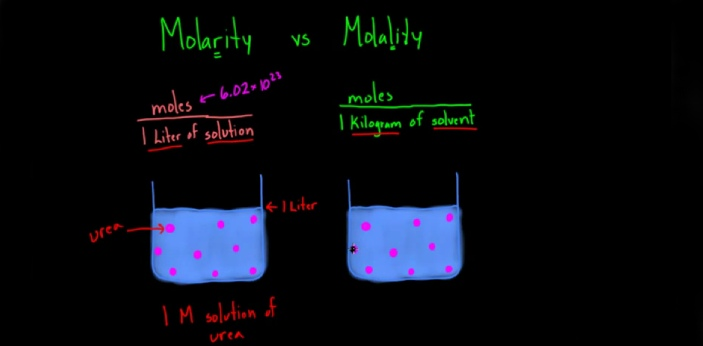What is the difference between Molarity and Molality? - ProProfs DiscussTopicsMoreProductsMore+ Ask Question

# What is the difference between Molarity and Molality?

Asked by R. Barnes, Last updated: Feb 26, 2020

###Request 0FollowShareAnswer AnonymouslyAnswer LaterCopy Link#### Matz Lewis Clark

Traveler and writer by profession.

Matz Lewis Clark, College student, Graduation, Orlando

Molarity and Molality are two terms which can be used to determine the concentration of a substance. Although it is very easy to mistake the two terms for each other, there are some differences between the two. Molarity can also be called Molar concentration. It is the concentration of a substance in solution, and it is calculated by dividing the number of moles of solute by one liter of solution.Molality, on the other hand, is also the concentration of a substance in solution, but it is expressed as the number of moles of solute per 1kg of the solvent. Molarity can be calculated by using moles/ liter, while Molality can be calculated using the formula, moles/kg. Change in temperature and pressure can affect the molarity of a substance, but Molality is unaffected by the change in pressure and temperature. Molarity is represented by the symbol 'M,' while Molality is represented by m'.#### A. Samuel

Writing quality content for contentment has been my passion since i was 21. I've been pursuing it as a Content Manager and Producer.

A. Samuel, Content Manager, Masters in Marketing and accounting, FloridaThe ‘‘Molarity’’ and ‘‘Molality’’ are measures of the concentration of a chemical solution. Molarity is also referred to as Molar concentration, and Molality is also referred to as Molal concentration. The following are the significant differences between Molarity and Molality: a. DEFINITION: Molarity is the ratio of moles of a substance (Solute) to the volume of the solution (mol/L) while Molality is the ratio of moles to the mass of the solvent (mol/kg). b. SYMBOL:Solutions labeled with the Molar concentration are denoted with a capital case ‘‘M’’ while solutions labeled with the Molal concentration are denoted with a lower case ‘‘m’’. c. PRECISION: Molarity may result in an imprecise and inaccurate concentration, while molality results in an accurate, precise measurement of concentration. d. FACTORS: Molarity is usually affected by the changes in pressure and temperature that occurs in the volume of solvent while Molality is generally unaffected by the changes in pressure and temperature since it is calculated based on mass and not volume.Search for Google imagesSelect a recommended image
Upload from your computerCancelSearch for Google imagesSelect a recommended image
Upload from your computerCancelSearch for Google imagesSelect a recommended image
Upload from your computerCancel